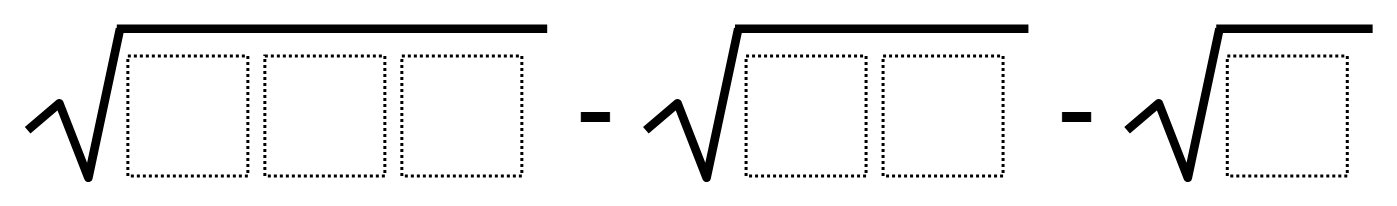Home > Grade 8 > Square Root Expression

# Square Root Expression

Directions: Use the digits 1 to 9, at most one time each, to fill in the boxes to make the following expression as close to 0 as possible.### Hint

What are all the perfect square three digit numbers? Several of these can’t be used since they contain a repeated digit (e.g. 121 uses the digit 1 twice and 144 uses the digit 4 twice)

### Answer

Using Perfect squares:
Sqrt(256) – sqrt(81) – sqrt(9) = 4

Using non-perfect squares:
Sqrt(145) – sqrt(92) – sqrt(6) = 0.00044178938368

Source: Erick Lee

## Triangle Sum Theorem

Directions: Using the digits 1-9 at most one time each, fill in the blanks so …

### One comment

1.Dang it.

I got:
√124 – √89 – √3 = -0.030503214